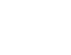• Developers
• < 1

## PHP and MATLAB Interfacing

• POSTED ON
• February 23, 2016
• POSTED BY
• Aayan Arif
• POSTED ON February 23, 2016
• POSTED BY Aayan Arif

In a project, the interfacing of PHP and MATLAB was required as client wanted the calculation of calories and quantity from a provided food image. Pre-requisites for this project were to have MATLAB installed on either Windows or Linux machine along with the Web server set up on the same machine. Before working on the actual project, we created a working demo....In a project, the interfacing of PHP and MATLAB was required as the client wanted the calculation of calories and quantity from a provided food image. Pre-requisites for this project were to have MATLAB installed on either Windows or Linux machine along with the Web server set up on the same machine. Before working on the actual project, we created a working demo for testing purposes.

In this demo, MATLAB file was doing calculations and saving the data in a file. The PHP script then executed this functionality and later on displayed the result of that particular file.

#### MATLAB Code:

The created MATLAB file was performing an addition operation. The result was saved in a result.txt file as:

```A = 5;
B = 5;
C = A + B;

fileID = fopen('result.txt','w');
fprintf(fileID,'%6.2fn',C);
fclose(fileID);```

#### PHP Code:

The PHP code given below will call MATLAB file to calculate and save the data in result.txt file.

Note: This is a very simple and short example, there can be better ways to write the same functionality.

Provided a 5 seconds delay to the file so that MATLAB code can write the output in result.txt file. Right after 5 seconds, the calculated result from result.txt file will be displayed.

```//This line will call MATLAB code
exec(""C:\Program Files\MATLAB\R2011a\bin\matlab.exe" -nodisplay -nosplash -nodesktop -r "run('C:\Users\Administrator\Desktop\myfile.m')"");

// 5 Seconds delay
sleep(5);
//Print Saved results from file
echo \$c;```

In this code, the PHP execution function was used to execute the .m file of MATLAB.  After that, a 5 seconds pause was set for MATLAB code processing. file_get_contents function would then get recently written content from result.txt file. In the end, the fetched result would be printed.